## 25 Questions MCQ Test Olympiad Preparation for Class 10 | Quadratic Equations - Olympiad Level MCQ, Class 10 Mathematics

Description
Attempt Quadratic Equations - Olympiad Level MCQ, Class 10 Mathematics | 25 questions in 25 minutes | Mock test for Class 10 preparation | Free important questions MCQ to study Olympiad Preparation for Class 10 for Class 10 Exam | Download free PDF with solutions
QUESTION: 1

Solution:
QUESTION: 2

### Solution of a quadratic equation x2+ 5x - 6 = 0

Solution: X^2 +5x-6=0
=x^2+6x-x-6=0[by splitting middle term]
=x(x+6)-1(x+6)
=(x-1)(x+6)
x=1;x=-6
QUESTION: 3

### If the sum of p terms of an A.P. is q and the sum of q terms is p, then the sum of (p + q) terms will be

Solution:

The sum of first p term of AP is q, which means that the number of terms is p

Thereby, let us take the first term as A and the common difference d

The sum of first q term of AP is p, which means that the number of terms is q

Thereby, let us take the first term as A and the common difference d

Therefore, the sum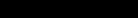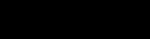Subtracting the sum of the term , p and q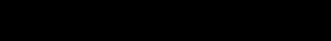After solving the equation we get the value of d as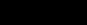Now with, first value of the series is a and the number of terms is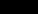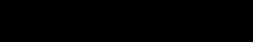Therefore, the sum isQUESTION: 4

If α,β are the roots of the equation x2 + 2x + 4 = 0, then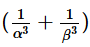is equal to :

Solution:
QUESTION: 5

If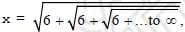then :

Solution:
QUESTION: 6

If α, β are the roots of the equation x2 + 7x + 12 = 0, then the equation whose roots are (α + β)2 and (α – β)2 is:

Solution:
QUESTION: 7

The value of k (k > 0) for which the equations x2 + kx + 64 = 0 and x2 – 8x + k = 0 both will have real roots is :

Solution:

Let D₁ and D₂ be the discriminant of the given equations and these will have equal roots only if D₁, D₂ ≥ 0
Or, if D₁ = (k² -4 × 64) ≥ 0  and D₂ = (64 - 4k) ≥ 0
Or,  if k² ≥ 256  and  4k ≤ 64
Or, if k ≥ 16  and  k ≤ 16
Or, k = 16
Hence the positive value of k is 16.

QUESTION: 8

If α,β are roots of the quadratic equation x2 + bx – c = 0, then the equation whose roots are b and c is

Solution:
QUESTION: 9

Solve for y : 9y4 – 29y2 + 20 = 0

Solution:
QUESTION: 10

Solve for x : x6 – 26x3 – 27 = 0

Solution:
QUESTION: 11

If 7th and 13th term of an A.P. are 34 and 64 respectively, then its 18th term is

Solution:
QUESTION: 12

Solve :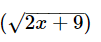–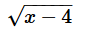= 3

Solution:
QUESTION: 13

Solve for x :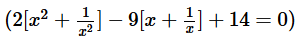:

Solution:
QUESTION: 14

Solve x :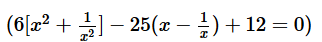:

Solution:
QUESTION: 15

Solve for x :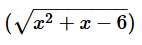– x + 2 =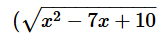, x ε R :

Solution:
QUESTION: 16

Solve for x : 3x+2 + 3-x = 10

Solution:
QUESTION: 17

Solve for x : (x + 1) (x + 2) (x + 3) (x + 4) = 24 (x ε R) :

Solution:
QUESTION: 18

The sum of all the real roots of the equation |x – 2|2 + |x – 2| – 2 = 0 is :

Solution:
QUESTION: 19

If a, b ε {1, 2, 3, 4}, then the number of quadratic equations of the form ax2 + bx + 1 = 0, having real roots is :

Solution:
QUESTION: 20

The number of real solutions of x –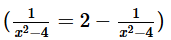is :

Solution:
QUESTION: 21

If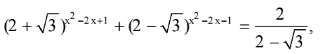then x is equal to :

Solution:
QUESTION: 22

The quadratic equation 3x2 + 2(a2 + 1) x + a2 – 3a + 2 = 0 possesses roots of opposite sign then a lies in:

Solution:
QUESTION: 23

The equation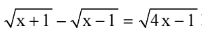has :

Solution:
QUESTION: 24

The number of real solutions of the equation 2|x|2 – 5|x| + 2 = 0 is :

Solution:
QUESTION: 25

The number of real roots of the equation (x – 1)2 + (x – 2)2 + (x – 3)2 = 0 :

Solution:Use Code STAYHOME200 and get INR 200 additional OFF Use Coupon Code